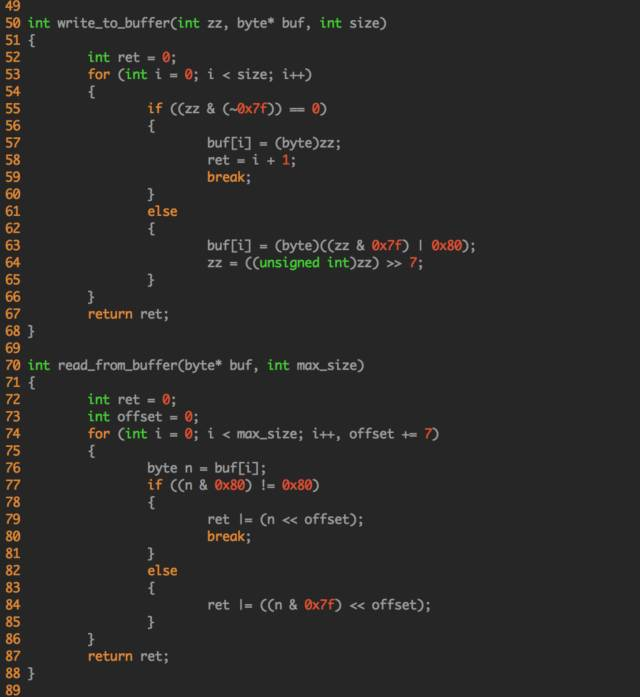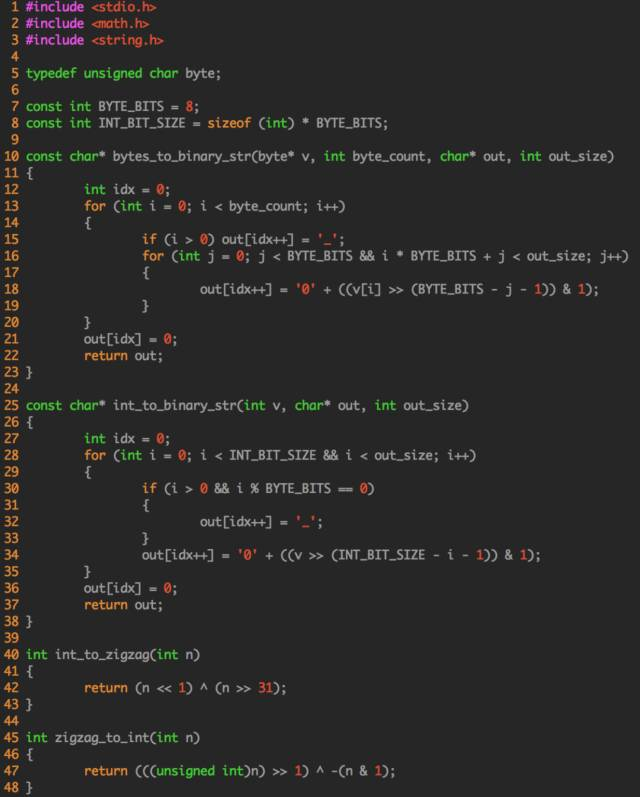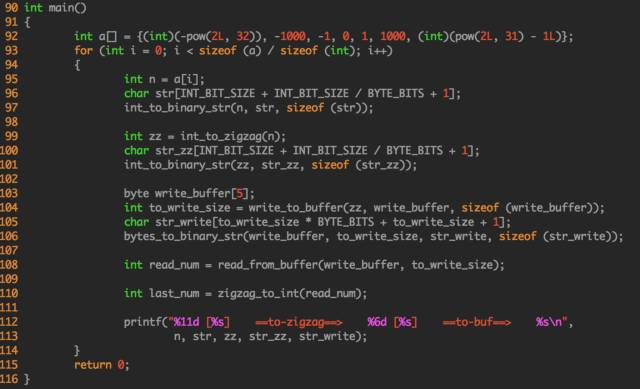# 压缩算法zigzag

## 小而巧的数字压缩算法：zigzag¶

1**、进制**

2**、补码**

A、原码

[+8] = 原

[-8] = 原

B、反码

[+8] = 原 = [0000 1000]反

[-8] = 原 = [1111 0111]反

1 + （-1）

= 原 + [1000 0001]原

= 原

= -2

1 + （-1）

= 反 + [1111 1110]反

= [1111 1111]反

= -0

C、补码

[+8] = 原 = [0000 1000]补

[-8] = 原 = [1111 1000]反

1 + （-1）

= 补 + [1111 1111]补

= [0000 0000]补

= 0

3**、zigzag**

zigzag给出了一个很巧的方法：我们之前讲补码讲过，补码的第一位是符号位，他阻碍了我们对于前导0的压缩，那么，我们就把这个符号位放到补码的最后，其他位整体前移一位：

(-1)10

=(11111111_11111111_11111111_11111111)补

=(11111111_11111111_11111111_11111111)符号后移

(-1)10

= (11111111_11111111_11111111_11111111)补

= (11111111_11111111_11111111_11111111)符号后移

= (00000000_00000000_00000000_00000001)zigzag

(1)10

= (00000000_00000000_00000000_00000001)补

= (00000000_00000000_00000000_00000010)符号后移

= (00000000_00000000_00000000_00000010)zigzag

int int_to_zigzag(int n)

{

​ return (n << 1) ^ (n >> 31);

}

n << 1 :将整个值左移一位，不管正数、0、负数他们的最后一位就变成了0；

(1)10

= (00000000_00000000_00000000_00000001)补

(-1)10

= (11111111_11111111_11111111_11111111)补

n >> 31: 将符号位放到最后一位。如果是非负数，则为全0；如果是负数，就是全1。

(1)10

= (00000000_00000000_00000000_00000001)补

(-1)10

= (11111111_11111111_11111111_11111111)补

(1)10 =>

(00000000_00000000_00000000_00000010)补 ^

(00000000_00000000_00000000_00000000)补

= (00000000_00000000_00000000_00000010)补

(-1)10 =>

(11111111_11111111_11111111_11111110)补 ^

(11111111_11111111_11111111_11111111)补

= (00000000_00000000_00000000_00000001)补

zigzag值还原为整型值：

int zigzag_to_int(int n)

{

​ return (((unsigned int)n) >> 1) ^ -(n & 1);

}

A、我们可以额外增加一个字节，用来表示接下来有效的字节长度，比如：00000001_00000010,前8位表示接下来有1个字节需要传输，第二8位表示真正的数据。这种方式虽然能达到我们想要的效果，但是莫名的增加一个字节的额外浪费。有没有不浪费的办法呢？

B、字节自表示方法。zigzag引入了一个方法，就是用字节自己表示自己。具体怎么做呢？我们来看看代码：

int write_to_buffer(int zz, byte* buf, int size)

{

​ int ret = 0;

​ for (int i = 0; i < size; i++)

​ {

​ if ((zz & (~0x7f)) == 0)

​ {

​ buf[i] = (byte)zz;

​ ret = i + 1;

​ break;

​ }

​ else

​ {

​ buf[i] = (byte)((zz & 0x7f) | 0x80);

​ zz = ((unsigned int)zz)>> 7;

​ }

​ }

​ return ret;

}

(~0x7f)16

=(11111111_11111111_11111111_10000000)补

(-1000)10

= (11111111_11111111_11111100_00011000)补

= (00000000_00000000_00000111_11001111)zigzag

(0000-0000000-0000000-0001111-1001111)zigzag

A、他跟(~0x7f)做与操作的结果，高位还有信息，所以，我们把低7位取出来，并在倒数第八位上补一个1(0x80)：11001111

B、将这个数右移七位：(0000-0000000-0000000-0000000-0001111)zigzag

C、再取出最后的七位，跟(~0x7f)做与操作，发现高位已经没有信息了（全是0），那么我们就将最后8位完整的取出来：00001111，并且跳出循环，终止算法；

D、最终，我们就得到了两个字节的数据[11001111, 00001111]

{

​ int ret = 0;

​ int offset = 0;

​ for (int i = 0; i < max_size; i++, offset += 7)

​ {

​ byte n = buf[i];

​ if ((n & 0x80) != 0x80)

​ {

​ ret |= (n <<offset);

​ break;

​ }

​ else

​ {

​ ret |= ((n & 0x7f) << offset);

​ }

​ }

​ return ret;

}

4、总结不过，在绝大多数情况下，小整数还是占主导的，所以整个算法才有了使用的基础。

（注：这个代码是老王自己改写的，自己review了，貌似没有错误。如果大家觉得写的有问题，请指正。）如果觉得c语言代码看起来不是很舒服，也可以去看thrift中java的源代码~